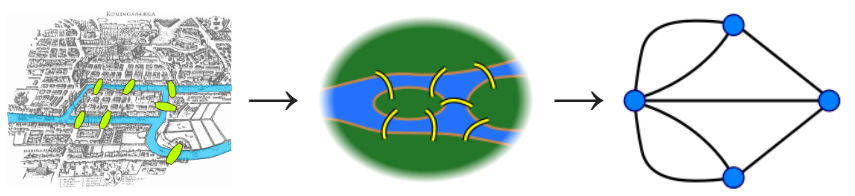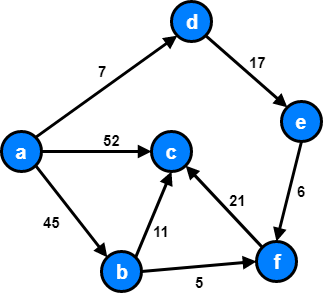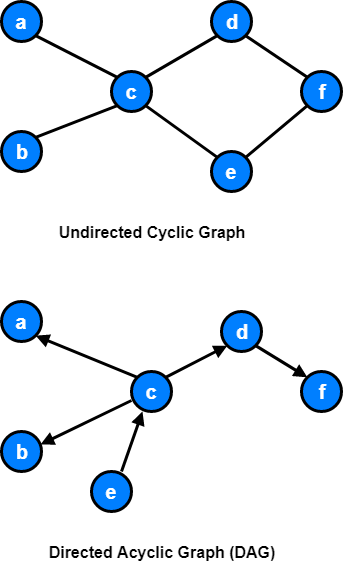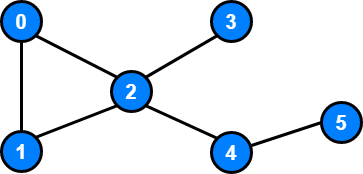# Graphs

Graph theory is a branch of Mathematics, first introduced in 1736 when mathematician Carl Ehler introduced Leonhard Euler to the Seven Bridges of Königsberg problem1.

The Seven Bridges of Königsberg problem is based in the former German city of Königsberg (now Kaliningrad, Russia) which lays on both sides of the Pregel River.

In the center, there were two large islands which were connected to each other and to the riverbanks by seven bridges. Carl Ehler became obsessed with finding a route to walk through each of the seven bridges without walking over any of them more than once.

Ehler reached out to Leonhard Euler, a Swiss mathematician. Euler confirmed Ehler’s hypothesis that the problem did not have a solution, and Euler’s explanation informed a new mathematical paradigm called Geometry of Position.

Euler’s new geometry paradigm stated that the location of the bridges didn’t matter. The problem, instead, can be simplified by turning each bridge into a point (node) with lines (edges) to represent links between them. This practice of using nodes and edges is now known as Graph Theory.## Terminology

Graph theory, like any topic, has many specific terms for aspects of a graph.Wow! A lot of jargon. Lets try to understand what all of these terms mean.

### Vertex

A vertex (also called a node) is a fundamental part of a graph. It can have a name (a key), it may also have additional information (the payload).

Our graph has 6 vertices:

`V = {a, b, c, d, e, f}`

### Edge

An edge (also called an “arc”) is another fundamental part of a graph. An edge connects two vertices to show that there is a relationship between them.

Our graph has 8 edges:

`E = {(a, b, 45), (a, c, 52), (a, d, 7), (b, c, 11), (b, f, 5), (d, e, 17), (e, f, 6), (f, c, 21)}`

### Directed or Undirected

In directed graphs, edges point from the node at one end to the node at the other end. In undirected graphs, the edges simply connect the nodes at each end.

Our example is a directed graph.

### Cyclic or Acyclic

A graph is cyclic if it has a cycle, an unbroken series of nodes with no repeating nodes or edges that connects back to itself. Graphs without cycles are acyclic.

Our example is a acyclic graph.### Weighted or Unweighted

If a graph is weighted, each edge has a “weight.” The weight could, for example, represent the distance between two locations, or the cost or time it takes to travel between the locations.

## Representing Graphs in Code

There are a few ways to represent graphs in the code. We’ll look at the most common three, and the basic tradeoffs.

Let’s take this graph as an example:### Edge List

A list of all the edges in the graph:

``````graph = [[0, 1], [0, 2], [1, 2], [2, 3], [2, 4], [4, 5]]
``````

Since node 0 has edges to nodes 1 and 2, `[0, 1]` and `[0, 2]` are in the edge list.

This is well suited to performant lookups of an edge, or listing all edges, but is slow with many other query types. For example, to find all vertices adjacent to a given vertex, every edge must be examined.

A list where the index represents the node and the value at that index is a list of the node’s neighbors:

``````graph = [
[1, 2],
[0, 2],
[0, 1, 3, 4],
,
[2, 5],

]
``````

Since node 2 has edges to nodes 0, 1, 3 and 4, `graph` has the adjacency list `[0, 1, 3, 4]`.

We could also use a dictionary where the keys represent the node and the values are the lists of neighbors.

``````graph = {
0: [1, 2],
1: [0, 2],
2: [0, 1, 3, 4],
3: ,
4: [2, 5],
5: 
}
``````

This would be useful if the nodes were represented by strings, objects, or otherwise didn’t map cleanly to list indices.

This representation allows for constant-time lookup of adjacent vertices, which is useful in many query and pathfinding scenarios.

It is slower for edge lookups, as the whole list of vertices adjacent to `u` must be examined for `v`, in order to find edge `uv`.

Adjacency lists are the typical choice for general purpose use, though edge lists or adjacency matrices have their own strengths, which may match a specific use case.

A matrix of 0 and 1 indicating whether node x connects to node y (0 means no, 1 means yes).

``````graph = [
[0, 1, 1, 0, 0, 0],
[1, 0, 1, 0, 0, 0],
[1, 1, 0, 1, 1, 0],
[0, 0, 1, 0, 0, 0],
[0, 0, 1, 0, 0, 1],
[0, 0, 0, 0, 1, 0]
]
``````

Since node 4 has edges to nodes 2 and 5, `graph` and `graph` have value 1.

Adjacency matrices perform strongly with edge lookups, with a constant-time lookup given a pair of vertex `Id`. They tend to be slow for other operations. For example, listing everything adjacent to a vertex requires checking every single vertex in the graph.

They also typically require more space than other models, especially with sparse graphs (graphs with “few” edges).

## Implementation

Using dictionaries, it is easy to implement the adjacency list in Python.

``````class Vertex:
def __init__(self, key):
self.id = key
self.connectedTo = {}

self.connectedTo[nbr] = weight

def __str__(self):
return str(self.id) + ' connected to: ' + str([x.id for x in self.connectedTo])

def get_connections(self):
return self.connectedTo.keys()

def get_id(self):
return self.id

def get_weight(self, nbr):
return self.connectedTo[nbr]

class Graph:
def __init__(self):
self.vertList = {}
self.numVertices = 0

self.numVertices = self.numVertices + 1
new_vertex = Vertex(key)
self.vertList[key] = new_vertex
return new_vertex

def get_vertex(self, n):
if n in self.vertList:
return self.vertList[n]
else:
return None

def __contains__(self, n):
return n in self.vertList